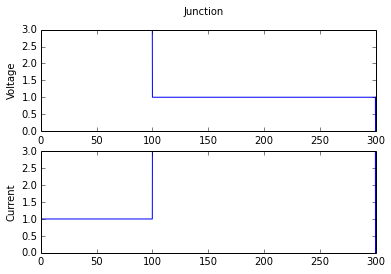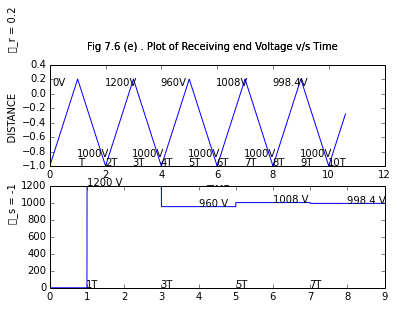# Chapter 7 : Transient Overvolatages and Insulation Coordination¶

## Example 7.1 Page No : 349¶

In :
 # GIVEN DATA
V = 1000. ; # surge voltage in kV
Z_c = 500. ; # surge impedance in Ω

# CALCULATIONS
# For case (a)
P = V**2/Z_c ; # Total surge power in MW

# For case (b)
V1 = V*10**3 ; # surge voltage in V
i = V1/Z_c ;# surge current in A

# DISPLAY RESULTS
print " a) Total surge power in line , P = %d MW "%P ;
print " b) Surge current in line , i = %d A "%(i) ;

 a) Total surge power in line , P = 2000 MW
b) Surge current in line , i = 2000 A


## Example 7.2 Page No : 350¶

In :
import math

# GIVEN DATA
V = 1000. ; # surge voltage in kV
Z_c = 50. ; # surge impedance in Ω

# CALCULATIONS
# For case (a)
P = V**2/Z_c ; # Total surge power in MW

# For case (b)
V1 = V*10**3 ; # surge voltage in V
i = V1/Z_c ;# surge current in A

# DISPLAY RESULTS
print " a) Total surge power in line , P = %d MW "%(P) ;
print " b) Surge current in line , i = %d A "%(i) ;

 a) Total surge power in line , P = 20000 MW
b) Surge current in line , i = 20000 A


## Example 7.4 Page No : 356¶

In :
import math

# GIVEN DATA
R = 500. ; # resistance in Ω
Z_c = 400. ; # characteristic impedance in Ω
v_f = 5000. ; # Forward travelling voltage wave in V
i_f = 12.5 ; # Forward travelling current wave in A

# CALCULATIONS
# For case (a)
r_v = (R - Z_c)/(R + Z_c) ; # Reflection coefficient of voltage wave

# For case (b)
r_i = -(R - Z_c)/(R + Z_c) ; # Reflection coefficient of current wave

# For case (c)
v_b = r_v * v_f ; # Backward-travelling voltage wave in V

# For case (d)
v = v_f + v_b ; # Voltage at end of line in V
v1 = (2 * R/(R + Z_c)) * v_f ; # (or) Voltage at end of line in V

# For case (e)
t1 = (2 * R/(R + Z_c)) ; # Refraction coefficient of voltage wave

# For case (f)
i_b = -( v_b/Z_c ) ; # backward-travelling current wave in A
i_b1 = -r_v * i_f ; # (or) backward-travelling current wave in A

# For case (g)
i = v/R ; # Current flowing through resistor in A

# For case (h)
t2 = (2 * Z_c/(R + Z_c)) ; # Refraction coefficient of current wave

# DISPLAY RESULTS
print ("EXAMPLE : 7.4 : SOLUTION :-") ;
print " a) Reflection coefficient of voltage wave , ρ = %.4f "%(r_v) ;
print " b) Reflection coefficient of current wave , ρ = %.4f "%(r_i) ;
print " c) Backward-travelling voltage wave , v_b = %.3f V "%(v_b) ;
print " d) Voltage at end of line , v = %.3f V "%(v) ;
print "     From alternative method "
print "     Voltage at end of line , v = %.3f V "%(v) ;
print " e) Refraction coefficient of voltage wave , Γ = %.4f "%(t1) ;
print " f) Backward-travelling current wave , i_b = %.4f A "%(i_b) ;
print " g) Current flowing through resistor, i = %.4f A "%(i) ;
print " h) Refraction coefficient of current wave , Γ = %.4f "%(t2) ;

EXAMPLE : 7.4 : SOLUTION :-
a) Reflection coefficient of voltage wave , ρ = 0.1111
b) Reflection coefficient of current wave , ρ = -0.1111
c) Backward-travelling voltage wave , v_b = 555.556 V
d) Voltage at end of line , v = 5555.556 V
From alternative method
Voltage at end of line , v = 5555.556 V
e) Refraction coefficient of voltage wave , Γ = 1.1111
f) Backward-travelling current wave , i_b = -1.3889 A
g) Current flowing through resistor, i = 11.1111 A
h) Refraction coefficient of current wave , Γ = 0.8889


## Example 7.5 Page No : 361¶

In :
%matplotlib inline
import math
from numpy import array,zeros,arange
from matplotlib.pyplot import plot,xlabel,ylabel,suptitle,subplot

# GIVEN DATA
Z_c1 = 400. ; # Surge impedance of line in Ω
Z_c2 = 40. ; # Surge impedance of cable in Ω
v_f = 200. ; # Forward travelling surge voltage in kV

# CALCULATIONS
# For case (a)
v_f1 = v_f * 10**3 ; # surge voltage in V
i_f = v_f1/Z_c1 ; # Magnitude of forward current wave in A

# For case (b)
r = (Z_c2 - Z_c1)/(Z_c2 + Z_c1) ; # Reflection coefficient

# For case (c)
t = 2 * Z_c2/(Z_c2 + Z_c1) ; # Refraction coefficient

# For case (d)
v = t * v_f ; # Surge voltage transmitted forward into cable in kV

# For case (e)
v1 = v * 10**3 ; # Surge voltage transmitted forward into cable in V
I = v1/Z_c2 ; # Surge current transmitted forward into cable in A

# For case (f)
v_b = r * v_f ; # surge voltage reflected back along overhead line in kV

# For case (g)
i_b = -r * i_f ; # surge current reflected back along overhead line in A

# For case (h)
# Arbitrary values are taken in graph.Only for reference not for scale
T = arange(0,300.1,0.1)     #T = 0:0.1:300 ;
vo = zeros(len(T))
for i in range(0,len(T)/3):  # plotting Voltage values
vo[i] = 3;

for i in range(len(T)/3,len(T)):
vo[i] = 1 ;

vo[i-1]=0;
'''
for i = int(length(T))
vo(i) = 0 ;
end
'''

subplot(2,1,1) ;
plot(T,vo)#,2)#,'012')#,'',[0,0,310,6]) ;
ylabel("Voltage")
suptitle("Junction")

io = zeros(len(T))
for i in range(0,len(T)/3):  # plotting Voltage values
io[i] = 1;

for i in range(len(T)/3,len(T)):
io[i] = 3 ;

io[i-1]=0;

subplot(2,1,2) ;
plot(T,io)#,5,'012','',[0,0,310,6]) ;
ylabel("Current")

# DISPLAY RESULTS
print ("EXAMPLE : 7.5 : SOLUTION :-") ;
print " a) Magnitude of forward current wave , i_f = %d A "%(i_f) ;
print " b) Reflection coefficient , ρ = %.4f "%(r) ;
print " c) Refraction coefficient , Γ = %.4f "%(t) ;
print " d) Surge voltage transmitted forward into cable , v = %.2f kV "%(v) ;
print " e) Surge current transmitted forward into cable , i = %.f A "%(I) ;
print " f) Surge voltage reflected back along the OH line , v_b = %.2f kV "%(v_b) ;
print " g) Surge current reflected back along the OH line , i_b = %.f A "%(i_b) ;
print " h Graph shows plot of voltage & current surges after arrival at the junction " ;

EXAMPLE : 7.5 : SOLUTION :-
a) Magnitude of forward current wave , i_f = 500 A
b) Reflection coefficient , ρ = -0.8182
c) Refraction coefficient , Γ = 0.1818
d) Surge voltage transmitted forward into cable , v = 36.36 kV
e) Surge current transmitted forward into cable , i = 909 A
f) Surge voltage reflected back along the OH line , v_b = -163.64 kV
g) Surge current reflected back along the OH line , i_b = 409 A
h Graph shows plot of voltage & current surges after arrival at the junction## Example 7.6 Page No : 366¶

In :
%matplotlib inline
import math
from numpy import arange,zeros
from matplotlib.pyplot import plot,subplot,xlabel,ylabel,text,show

# GIVEN DATA
v = 1000 ; # ideal dc voltage source in V
Z_s = 0. ; # internal impedance in Ω
Z_c = 40. ; # characteristic impedance in Ω
Z_r = 60. ; # Cable is terminated in 60Ω resistor

# CALCULATIONS
# For case (a)
r_s = (Z_s - Z_c)/(Z_s + Z_c) ; # Reflection coefficient at sending end

# For case (b)
r_r = (Z_r - Z_c)/(Z_r + Z_c) ; # Reflection coefficient at receiving end

# For case (c)
T = arange(0,10.601,0.001)      #0:0.001:10.6 ; # # plotting values
x = zeros(len(T))
for i in range(len(T)):
if(T[i]<=1):
x[i] = (1.2)*T[i] - 1 ;
elif(T[i]>=1 and T[i]<=2):
x[i] = (-1.2)*T[i] + 1.4 ;
elif(T[i]>=2 and T[i]<=3):
x[i] = (1.2)*T[i]- 3.4 ;
elif(T[i]>=3 and T[i]<=4):
x[i] = (-1.2)*T[i] + 3.8 ;
elif(T[i]>=4 and T[i]<=5):
x[i] = (1.2)*T[i]- 5.8 ;
elif(T[i]>=5 and T[i]<=6):
x[i] = (-1.2)*T[i] + 6.2 ;
elif(T[i]>=6 and T[i]<=7):
x[i] = (1.2)*T[i]- 8.2 ;
elif(T[i]>=7 and T[i]<=8):
x[i] = (-1.2)*T[i] + 8.6 ;
elif(T[i]>=8 and T[i]<=9):
x[i] = (1.2)*T[i]- 10.6 ;
elif(T[i]>=9 and T[i]<=10):
x[i] = (-1.2)*T[i] + 11 ;
elif(T[i]>=10 and T[i]<=10.6):
x[i] = (1.2)*T[i] - 13 ;

subplot(2,1,1) ; # Plotting two graph in same window
plot(T,x)#,5,'012','',[0,-1,11,0.2]) ;

xlabel("TIME") ;
ylabel(u"ρ_s = -1             DISTANCE             ρ_r = 0.2") ;
suptitle("Fig 7.6 (c) Lattice diagram") ;
#xset('thickness',2) ; # sets thickness of axes
text(1,-1,u'T') ;
text(2,-1,u'2T') ;
text(3,-1,u'3T') ;
text(4,-1,u'4T') ;
text(5,-1,u'5T') ;
text(6,-1,u'6T') ;
text(7,-1,u'7T') ;
text(8,-1,u'8T') ;
text(9,-1,u'9T') ;
text(10,-1,u'10T') ;
text(0.1,0.1,u'0V') ;
text(2,0.1,u'1200V') ;
text(4,0.1,u'960V') ;
text(6,0.1,u'1008V') ;
text(8,0.1,u'998.4V') ;
text(1,-0.88,u'1000V') ;
text(3,-0.88,u'1000V') ;
text(5,-0.88,u'1000V') ;
text(7,-0.88,u'1000V') ;
text(9,-0.88,u'1000V') ;

# For case (d)
q1 = v ; # Refer Fig 7.11 in textbook
q2 = r_r * v ;
q3 = r_s * r_r * v ;
q4 = r_s * r_r**2 * v ;
q5 = r_s**2 * r_r**2 * v ;
q6 = r_s**2 * r_r**3 * v ;
q7 = r_s**3 * r_r**3 * v ;
q8 = r_s**3 * r_r**4 * v ;
q9 = r_s**4 * r_r**4 * v ;
q10 = r_s**4 * r_r**5 * v ;
q11 = r_s**5 * r_r**5 * v ;
V_1 = v - q1 ;
V_2 = v - q3 ;
V_3 = v - q5 ;
V_4 = v - q7 ; # voltage at t = 6.5T and x = 0.25l in Volts
V_5 = v - q9 ;

# For case (e)
t = arange(0,9.001,0.001)     #t = 0:0.001:9 ;
y = zeros(len(t))
for i in range(len(t)):
if(t[i]>=0 and t[i]<=1):
y[i] = V_1 ;
elif(t[i]>=1 and t[i]<=3):
y[i] = V_2 ;
elif(t[i]>=3 and t[i]<=5):
y[i]= V_3 ;
elif(t[i]>=5 and t[i]<=7):
y[i]= V_4 ;
elif(t[i]>=7 and t[i]<=9):
y[i]= V_5 ;

subplot(2,1,2) ;
#a = gca() ;
#a.thickness = 2 ; # sets thickness of plot
plot(t,y)#,2,'012','',[0,0,10,1300]) ;
#a.x_label.text = 'TIME (T)' ; # labels x-axis
#a.y_label.text = 'RECEIVING-END   VOLTAGE (V)' ; # labels y-axis
suptitle("Fig 7.6 (e) . Plot of Receiving end Voltage v/s Time") ;
#xset('thickness',2); # sets thickness of axes
text(1,0,u'1T') ; # naming points
text(3,0,u'3T') ;
text(5,0,u'5T') ;
text(7,0,u'7T') ;
text(1,1200,u'1200 V') ;
text(4,960,u'960 V') ;
text(6,1008,u'1008 V') ;
text(8,998.4,u'998.4 V') ;
show()

# DISPLAY RESULTS
print ("EXAMPLE : 7.6 : SOLUTION :-") ;
print " a) Reflection coefficient at sending end , ρ_s = %.f "%(r_s) ;
print " b) Reflection coefficient at sending end , ρ_r = %.1f "%(r_r)
print " c The lattice diagram is shown in Fig 7.6 c " ;
print " d) From Fig 7.6 c) , the voltage value is at t = 6.5T and x = 0.25 l is = %.d Volts "%(V_4) ;
print " e The plot of the receiving-end voltage v/s time is shown in Fig 7.6 e " ;EXAMPLE : 7.6 : SOLUTION :-
a) Reflection coefficient at sending end , ρ_s = -1
b) Reflection coefficient at sending end , ρ_r = 0.2
c The lattice diagram is shown in Fig 7.6 c
d) From Fig 7.6 c) , the voltage value is at t = 6.5T and x = 0.25 l is = 1008 Volts
e The plot of the receiving-end voltage v/s time is shown in Fig 7.6 e